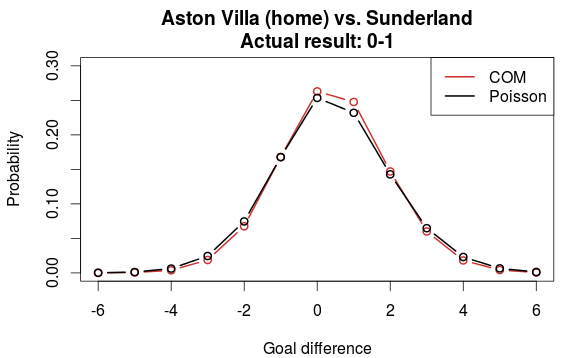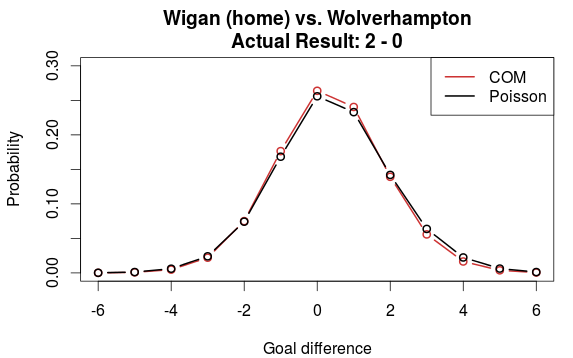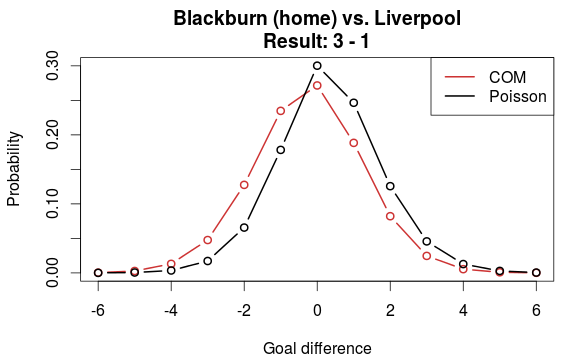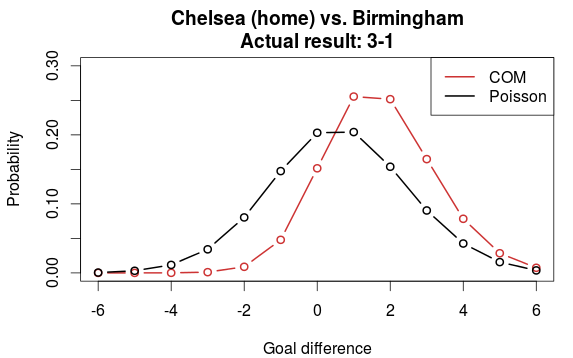# The underdispersed Conway-Maxwell Poisson distribution and goal differences

I have unfortunately not had the time to look more closely at the performance for the underdispersed count distributions that I in my last post found to be useful for predicting football results. Here I am taking a quick look into how the Conway-Maxwell distribution (COM) influences the predicted goal differences compared to the Poisson distribution.

Using data from the 2010-11 Premier League season I fitted the both the Poisson model and the COM model. The estimated dispersion parameter for the COM model indicated that there was less variability in the actual goals scored than implied by the Poisson distrubtion. I used the code I posted here to compute the probability distributions for the goal-differences for five matches in the season.

Let’s first look at the goal difference distribution for Arsenal playing at home against Manchester City. Both teams were in the top of the final table, and the actual result for this game in the 2010-11 season was 0-0. Comparing the distributions from the Poisson and COM models we see that they are pretty much identical.For Aston Villa vs. Sunderland, which placed 9th and 10th on the table in 2010-11, we also see that there is not much difference between the two models. Although there is a slight increase in the probability for the actual result in that game, I don’t think it is of much importance.Let’s compare the models using two teams from the bottom of the table, Wigan vs. Wolverhampton. Again, not much difference. Also note that there is basically no change in the probability for the actual result.OK, so far the comparisons have been based on teams of similar strengths. But take Blackburn (15th on the table) vs. Liverpool (6th). Now we see that the COM model and Poisson model differ a bit. Here the COM model does a worse prediction of the actual result compared to the Poisson model. But only considering the league positions, the skewing of the distribution in favor of Liverpool in this case may not be totally unreasonable.The last plot is compares the distributions between Chelsea (2nd on the table) vs. Birmingham (18th). Here we clearly see that there is a substantial difference in the prediction between the two models. The COM model favors Chelsea much more than the Poisson model does, which in this case give a much higher probability for the correct result.From just looking at these few plots, I think we can conclude that the (underdispersed) COM model differs from the Poisson model where there is a greater difference in strength between the two sides.

## 5 thoughts on “The underdispersed Conway-Maxwell Poisson distribution and goal differences”

1.Doc on said:

Not very scientific, but if you divide the population into 2 arbitrary sets, relatively equal (poison) , unequal (com) , do you get better results than purely evaluating poison or com on the entire set? Guess you would need some way to define a 2 step model

2.hm1103 on said:

Hi, how to apply CompGLM into this command?
probability_matrix <- dpois(0:maxgoal, lambda) %*% t(dpois(0:maxgoal, mu))

when I replaced dpois with dcomp, I need to input the nu value in dcomp formula. Also, how can I find out the nu value?

•opisthokonta on said:

The dcomp-function uses a parameterization other than the one I prefer. I prefer to use a function like this:

```dcomp2 <- function(y, mu, sigma, ...){
lamm <- mu^sigma
dcomp(y, lam=lamm, nu=sigma, ...)
}
```

Where mu is the location parameter (approximately the expected value, although it is a bit more complicated than that.) and sigma is the dispersion parameter.

To estimate the the sigma parameter you can use the optim function. This is the approach I have used in my implementation of the Dixon-Coles model: Make a function that calculates the sum of the log-probabilities of all your data for a given set of parameters (regression coefficients and other parameters such as the dispersion parameter). Use optim to find the parameter values that maximizes the log-probability.

I am also writing on a post with some more details about the comp-model.

Edit: Have you tried the glm.comp function? I haven’t, but it may be worth taking a look at.

3.MAK on said:

Hi Jonas, what represents a negative goal difference here? thank you

•opisthokonta on said:

A negative goal difference indicates that the away team scores more goals than the home team. Goal difference is home – away.